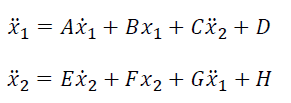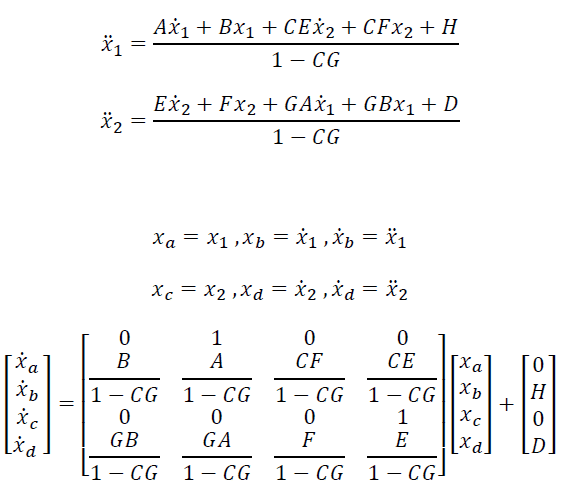# Solution to Coupled Second Order ODE's

## Homework Statement

[/B]
I'm trying to 'solve' two coupled second order ODE's with the intent of putting them in state space. My specific problem is more complex and includes additional equations which are irrelevant. Essentially I can solve the problem if I know the solution to this. x1 and x2 are time dependent variables and A-H are constant coefficients.

I initially thought I could simply plug x2 into x1's equation to obtain one equation and then x1 into x2's to obtain the other equation. However, I feel like this would create a redundant state space matrix which would be otherwise useless. Really all I need is these two in reduced state space

## Homework Equations[/B]

## The Attempt at a Solution#### Attachments

Last edited by a moderator:

Chestermiller
Mentor
This looks like a correct approach. Is there a question?

Incidentally, these are coupled ODEs, not PDEs.

Chet

Mark44
Mentor
Incidentally, these are coupled ODEs, not PDEs.
Now fixed

•Chestermiller
I was just wondering if this was a valid approach to state space from two second order equations to four first orders. I appreciate the input and if this is valid then all is well. Thanks! Ah yes , most definitely ODE's, I must have mis-typed the 'O'... twice.

Chestermiller
Mentor
I was just wondering if this was a valid approach to state space from two second order equations to four first orders. I appreciate the input and if this is valid then all is well. Thanks! Ah yes , most definitely ODE's, I must have mis-typed the 'O'... twice.
You should be made aware that there is a standard way of analytically solving a set of linear coupled first order ODEs like this.

You should also be made aware that the method you so cleverly developed so far (no sarcasm intended) is also a standard method of analyzing coupled higher order ODEs. Welcome to the world of "reinventing the wheel." I've personally visited that world lots of times.

Chet

•Hypercube